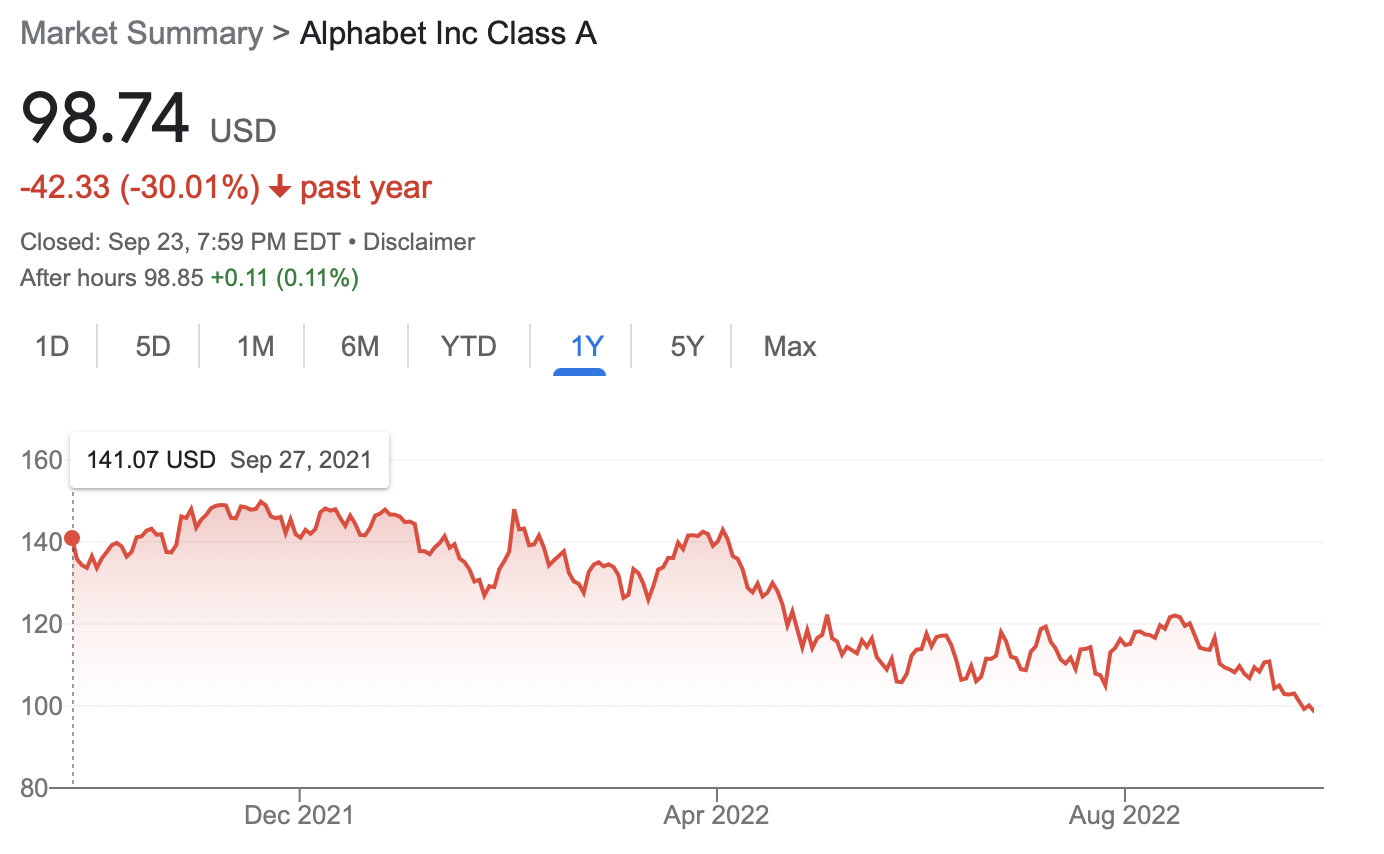# Lecture 22: Intro to Dynamic Programming

## Announcements

1. ‘22E Honors Thesis talks Today!
• 4-5pm in SCCE A131
1. Courses Next Semester

• 225 Algorithms and Visualization
• 273 Parallel and Distributed Computing

## Overview

1. Memoization
2. Profit Maximization, Revisited

## So Far

1. Divide and Conquer

2. Greedy

And now…

## Features of Dynamic Programming

1. Break problem into smaller sub-problems
2. Iterate over sub-problems to produce solution

Compare to divide and conquer:

1. Break problem into smaller sub-problems
2. Recursively solve sub-problems
3. Combine solutions

## Issues with Recursion

Recall the Fibonacci Sequence:

• $1, 1, 2, 3, 5, 8, 13, 21, 34, 55, \ldots$

Defined by:

1. $f(1) = f(2) = 1$
2. for $n > 2$, $f(n) = f(n-1) + f(n-2)$

Recursive code:

  Fib(n):
if n <= 2 then return 1
return Fib(n-1) + Fib(n-2)


## What is Running Time?

  Fib(n):
if n <= 2 then return 1
return Fib(n-1) + Fib(n-2)


## Redundant Recursive Calls

Recursive subproblems overlap

## Memoization

Recursing without recursion

• store results of recursive calls
• iterate over results rather than making recursive calls
• compute results “bottom up” rather than “top down”
• iterate over stored values to compute “next” value

How to do for Fibonacci?

## Memoized Fibonacci

  MFib(n):
a <- array of size n
a <- 1
a <- 1
for each index i from 3 to n do
a[i] <- a[i-1] + a[i-2]
endfor
return a[n]


## Running Time?

  MFib(n):
a <- array of size n
a <- 1
a <- 1
for each index i from 3 to n do
a[i] <- a[i-1] + a[i-2]
endfor
return a[n]


## The Moral

With memoization, we converted

• recursive procedure
• many redundant recursive calls
• running time $\Omega(2^{n/2})$

into

• iterative procedure
• running time $O(n)$

# Profit Maximization

## Recall: Profit MaximizationGoal. Pick day $b$ to buy and day $s$ to sell to maximize profit.

## Formalizing the Problem

Input. Array $a$ of size $n$

• $a[i] =$ price of Alphabet stock on day $i$

Output. Indices $b$ (buy) and $s$ (sell) with $1 \leq b \leq s \leq n$ that maximize profit

• $p = a[s] - a[b]$

## Divide and Conquer Algorithm

  MaxProfit(a, i, j):
if j - i = 1 then return 0
m <- (i + j) / 2
left <- MaxProfit(a, i, m)
right <- MaxProfit(a, m, j)
min <- FindMin(a, i, m)
max <- FindMax(a, m, j)
return Max(left, right, max - min)


Running time?

## Another (Recursive) Procedure?

• consider last day, $n$
• two cases for optimal solution:
1. max profit achieved by selling on day $n$
2. max profit achieved by selling before day $n$

Questions.

1. In case 1, how should we determine buy date?

2. In case 2, how should we compute max profit?

## Recursive Procedure

  MaxProfit(a, n):
if n = 1 then return 0
min <- FindMin(a, n)
max <- MaxProfit(a, n-1)
return max(a[n] - min, max)


Running time?

## Questions

1. What makes MaxProfit inefficient?

2. What part(s) of the procedure can be memoized?

## Memoizing MaxProfit

Create two arrays:

1. min[i] stores minimum value in a[1..i]
2. max[i] stores maximum profit achievable by selling up to time i

Question. How to update these arrays?

## Memoized Maximum Profit

  MMaxProfit(a):
initialize arrays min, max
min <- a
max <- 0
for i from 2 to n do
min[i] <- Min(min[i-1], a[i])
max[i] <- Max(max[i-1], a[i] - min[i])
endfor
return max[n]


## Correctness

Induction on $n$…

## Running Time?

  MMaxProfit(a):
initialize arrays min, max
min <- a
max <- 0
for i from 2 to n do
min[i] <- Min(min[i-1], a[i])
max[i] <- Max(max[i-1], a[i] - min[i])
endfor
return max[n]


## Optimization

Can do without arrays for min and max

  MMaxProfit(a):
min <- a
max <- 0
for i from 2 to n do
min <- Min(min, a[i])
max <- Max(max, a[i] - min)
endfor
return max[n]


## Exercise

Update MMaxProfit to return the buy/sell days in addition to the maximum achievable profit.

## Next Time

• weighted interval scheduling
• much more!